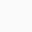# square tricks of numbers above and below 50| Mathematics Tricks

Finding square of two digit numbers (41 to 59) is very tricky and easy. Here it has been shown to find the square of numbers below(upto 41) and above 50 (upto 59) . This trick holds true only in this range.
First we find the square of 50 .
50^2=2500
we take the above square as reference to calculate the square of the number below 50 and afoul 50.
case I: Here are calculate the square of 48 .
As 48 is less than 50 by’ 2′ . therefore me simply can write the square of 48 without doing calculation.
48^2 =2304
Here we subtracted 2 from 25 to get 2300. Next we add 2^2 to 2300 ; which becomes 2304 .
46^2 =2116
Here we subtracted 4 from 25 to get 2100. Next we add 4^2 to 2100 ; which becomes 2116 .
53^2= 2809 .
Here we add 3 to 25 to get 2800. Next we add 3^2 to 2800 ; which becomes 2809 .

##### One thought on “square tricks of numbers above and below 50| Mathematics Tricks”
1.anky says:

I appreciate your tricks and this will be very helpful in saving our time specially in simplification kind of problems.
Solving Rational Numbers